﻿ Analytical Modeling of a Piezoelectric Bimorph Beam

### Analytical Modeling of a Piezoelectric Bimorph Beam

A. Lebied, B. Necib, M. SAHLI

## Analytical Modeling of a Piezoelectric Bimorph Beam

A. Lebied1,, B. Necib1, M. SAHLI2

1Mechanical Engineering Department, Faculty of Technology Sciences, University Constantine 1, Algeria

2Departement de Mécanique Appliquée, ENSMM, 24 chemin de l’Epitaphe, 25030 Besançon, France

### Abstract

Smart structures based on piezoelectric materials are now finding applications in a wide variety of environmental conditions. Such materials are capable of converting mechanical energy into electrical energy. Indeed, when subjected to mechanical stress become electrically charged at their surface and vice versa. In the current paper, the research is focused on a simple analytical model based on Euler–Bernoulli beam theory with the following assumptions was proposed: (a) the piezoelectric layer thickness in comparison to the length of the beam is very thin and (b) the electrical field between the upper surface and lower surface of the piezoelectric layer is uniform. We have applied this model to study its static responses and predict the ambient deformations into usable electrical energy from a cantilever piezoelectric beam. The proposed model was numerically investigated for validity. Analytical data showed that the proposed model simulations are in good agreement with the FE results. The results of the modeling are very promising.

• A. Lebied, B. Necib, M. SAHLI. Analytical Modeling of a Piezoelectric Bimorph Beam. American Journal of Mechanical Engineering. Vol. 4, No. 1, 2016, pp 7-10. http://pubs.sciepub.com/ajme/4/1/2
• Lebied, A., B. Necib, and M. SAHLI. "Analytical Modeling of a Piezoelectric Bimorph Beam." American Journal of Mechanical Engineering 4.1 (2016): 7-10.
• Lebied, A. , Necib, B. , & SAHLI, M. (2016). Analytical Modeling of a Piezoelectric Bimorph Beam. American Journal of Mechanical Engineering, 4(1), 7-10.
• Lebied, A., B. Necib, and M. SAHLI. "Analytical Modeling of a Piezoelectric Bimorph Beam." American Journal of Mechanical Engineering 4, no. 1 (2016): 7-10.

 Import into BibTeX Import into EndNote Import into RefMan Import into RefWorks

123
Prev Next

### 1. Introduction

Piezoelectric materials, such as Lead Zirconate Titanate (PZT), have the ability to convert mechanical forces into an electric field in response to the application of mechanical stresses or vice versa [1, 2, 3]. This effect is due to the spontaneous separation of charge within certain crystal structures, thereby producing an electric dipole. For a piezoelectric material, it is known that the output voltage of the material is a function of stress. Typically, stress is achieved through the displacement or bending of the piezoelectric beam. These novel properties of the materials have found applications in sensor and actuator technologies, and recently, in the new field of energy harvesting [4, 5, 6, 7].Download asVeiw figureFigures index
Figure 1. Schematic illustration of the poling process

The origin of the piezoelectric effect is related to an asymmetry in the unit cell and the resultant generation of electric dipoles due to the mechanical distortion. These materials do not possess any piezoelectric properties owing to the random orientations of the ferroelectric domains in the ceramics before poling. During poling, an electric field is applied on the ferroelectric ceramic sample to force the domains to be oriented or poled. After poling, the electric field is removed and a remnant polarization and remnant strain are maintained in the sample, and the sample exhibits piezoelectricity. A simple illustration of the poling process is shown in Figure 1.

In recent years, there have been a considerable number of publications using various models for the electromechanical behavior of piezoelectric energy harvester beams, as can be seen in Anton and Sodano . Eggborn  developed the analytical models to predict the energy harvesting from a cantilever beam and a plate using Bernoulli-beam theory and made a comparison with the experimental result. Ajitsaria et al.  developed modeling and analysis of a bimorph piezoelectric cantilever beam for voltage generation using on the analytical approach based on Euler–Bernoulli beam theory and Timoshenko beam equations, which is then compared with two previously described models in the literature: the electrical equivalent circuit and energy method. Lead Zirconate titanate (PZT) is the most used piezoelectric material because of its high electromechanical coupling characteristics in single crystals [11, 12]. Sodano et al.  developed an analytical model of a beam with attached PZT elements to provide an accurate estimate of the power generated by the piezoelectric effect.

Erturk and Inman have recently published a series of papers on energy harvesting using the cantilever model and their provide a broad coverage of several important modeling aspects that were validated with experimental data [14, 15, 16, 17, 18].

Works presented in this report concerns the problems the characteristic phenomena of piezoelectricity. In the current paper, the research is focused on the different deformations effect by voltage generation of the piezoelectric beam. The relation between the voltage imposed and the curvature is derived which is used to explain the effect of placement on voltage generation is investigated. The predictive models are validated by being compared to numerical data.

### 2. Theory and System Modeling

Piezoelectricity involves the interaction between electrical and mechanical behaviors of the material. Meanwhile, the static linear relations between the electrical and mechanical variables can approximate this interaction. In strain-charge form, these relations can be given by the following equations: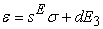(1)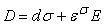(2)

Where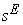,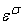and d are the elastic compliance coefficient, electric field related electrostriction coefficient and linear permittivity respectively and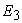are the electric field intensity .

In equations (1) and (2) above, the electrical quantities (E, D) have vector nature, while the mechanical quantities (σ, s) have tensor nature of six components. In piezoelectric materials, the constants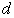, e and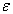depend on the directions of electric field, displacement, as well as stress and strain. The piezoelectric strain constant (d) can be presented as dij which can be interpreted as charge created in the ith direction under a stress applied in the jth direction.

The linear constitutive equations for a piezoelectric material  have been employed in terms of the piezoelectric coefficient e31 and the electric field applied across the thickness of the layer E3.

### 3. Cantilever Beam Analysis

The following section describes the development of the PZT models and the analytical estimations of local deformation. The schematic view of the beam undertaken in this study is shown in Figure 2. If the thickness of the both host substrate layers equal to 200µm, respectively hp1, hp2 are far greater than the thickness of the electrode patch (500nm), and the PZT beam length (L) equal 10mm is approximately the length of the electrode layer. The PZT beam width (w) equal 1mm is approximately thirty times the width of the electrode layer (w0=30µm, neglected in the analytical model proposed). A thin electrode layer was attached to the PZT layer using two-part epoxy glue in a typical bimorph configuration (see Figure 3). The PZT PCI-151 was used to manufacture the cantilever beam device. The dimensions and properties of the materials are shown in Table 1.

Based on piezoelectric cantilever, the most important piezoelectric strain coefficients when designing harvesting devices are d31 and d33 (Roundy et al., 2003). In the 3-1 mode, imposed strain in the 1-direction is perpendicular to the electric field in the piezoelectric material (i.e. in the 3-direction). In the 3-3 mode, the strain and electric field are parallel to each other (in the 3-direction).

The electrical–mechanical coupling of the 3-3 mode (or z-z mode) is higher than the 3-1 mode (or z-x mode) and the 3-3 mode piezoelectric cantilever will allow for a much higher open circuit voltage compared to a similarly sized 3-1 cantilever. Another key advantage of the 3-1 mode of operation over the 3-3 system is that the 3-1 system is much more compliant, hence larger strains can be produced with smaller input forces. The piezoelectric constitutive equation (2) can be rewritten as: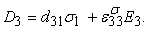(3)

#### Table 1. The Dimensions and Properties of Electrode and Pzt BeamDownload asPowerPoint Slide

Veiw figure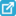View current table in a new windowDownload asVeiw figureFigures index
Figure 2. Schema a piezoelectric cantilever beam and electrode patchsDownload asVeiw figureFigures index
Figure 3. The attachment of electrode on the PZT beam

### 4. Analytical Results and Discussions

The modeling of a cantilever beam by means of the substitution potential V1, V2,V3, V4, by the two command variables of the beam axes Vy et Vz of such so that: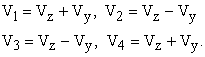The moment equilibrium equation along y is expressed by: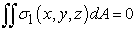(4)

The relative deformation of the beam due to the elasticity is given by: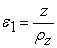(5)

Furthermore, the slope at an abscissa x of the beam is in a first approximation: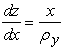(6)

The resolution of equation (4) by using equation (6) we obtain the following deflection z, δz: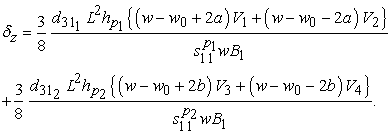With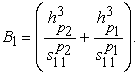Taking our case the two piezoelectric plates are the same, therefore if the centering error of the electrodes is the same on both faces of the bimorph, or a = b or a=-b.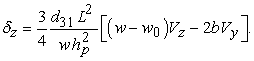Moreover, it is possible to draw on the same graph of the deflection curve of the bimorph beam obtained for different values of a and b errors. The superposition of these curves to quantify the value of the deflection at the end of the cantilever. It was found that this deflection is directly proportional to the value of the electric field Vy by setting the voltage Vz = 100V (cf.Figure 4).Download asVeiw figureFigures index
Figure 4. Deflections δz according to Vy for several centering errors as a=-b and Vz =100V

In this case we observe that for a = b = 0 there is a stabilization of the deflection for any value of the tension Vy against to Vy=100 V the results obtained without taking account the error values a and b (cf.Figure 5).

However, the values of a and b depend primarily on the technical means of realization implemented for the development of these actuators. From a technological point of view, it is difficult to strictly satisfy the condition a = b = 0, or even a = b.

Let us now try fixing the two tensions and traced the deflections based on centering errors electrodes a and b on the coupling in the case of a =-b.Download asVeiw figureFigures index
Figure 5. Deflections δz according to Vz for several centering errors as a=-b and Vy =100VDownload asVeiw figureFigures index
Figure 6. Deflections δz according to Vz for several centering errors as a=-b and Vy =100V

The Figure 6 presents the deflection as a function a=-b, for Vy = 100V and for several values of Vz. In these conditions, we observed not only a decrease of the deflection when a = b increases, but also a coupling Vz on δz.

### 5. FE Modeling Validation

As illustrated in Figure 7, the analytical solution in terms of the evolution of the deflection as a function of voltage conforms to the numerical simulation with the piezoelectric material cited below. The numerical curve being almost equivalent to the analytical.

We also assume that there is no centering error, or a = b = 0 We then obtain for a degree of freedom in z, the following equation: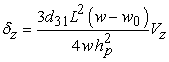(5)Download asVeiw figureFigures index
Figure 7. Free deflections δz of the piezoelectric beam depending on the control voltage Vz

### 6. Conclusion

Modeling the clamped-free piezoelectric beam small displacement was developed and presented in this article. The electromechanical coupling has been considered. The analytical results were valid for comparisons with numerical results find in the literature. The analytical results showing a good correlation and agree very well. It was also concluded that the actuator and the sensor will be better placed at underrun because it is the position or the actuator has the greatest impact and where the sensor gives the greatest signal. They are co-located as said Colles one below the other on either side of the beam.

This modeling is again applicable to structures of any shape and may include piezoelectric components to form a control or vibration. In addition, they allow us to know the magnitude of the force imposed equivalent to the applied field for a given displacement. These results are necessary for the design, optimization and development of systems for micromanipulation and assembly of micro objects.

### References

  S. Roundy, P.K. Wright, “A piezoelectric vibration based generator for wireless electronics, Smart Mater. Struct., Vol.13, pp.1131-1142, 2004.In article View Article  S.R. Anton, H.A. Sodano, “A review of Power harvesting using piezoelectric materials (2003-2006),” Smart Materials and Structures, Vol.16, pp.R1-R21, 2007.In article View Article  E. F. Crawley, J. de Luis, “Use of Piezoelectric Actuators as Elements of Intelligent Structures,” Present as Paper 86-0878 at the AIAA/ASME/ASCE/AHS Active Structures, Structural Dynamics and Materials Conference, San Antonio, TX, May 19-21, 1986.In article View Article  P. Muralt, “Ferroelectric thin films for micro-sensors and actuators: a review,” Journal of Micromechanics and Microengineering, Vol. 10, no. 2, pp. 136-146, 2000.In article View Article  C. Niezrecki, D. Brei, S. Balakrishnan, and A. Moskalik, “Piezoelectric actuation: state of the art,” Shock and Vibration Digest, Vol. 33, no. 4, pp. 269-280, 2001.In article View Article  J. F. Tressler, S. Alkoy, and R. E. Newnham, “Piezoelectric sensors and sensor materials,” Journal of Electroceramics, Vol. 2, no. 4, pp. 257-272, 1998.In article View Article  J. Ajitsaria, S.Y. Choe, D. Shen, D.J. Kim, “Modeling and analysis of a bimorph piezoelectric cantilever beam for voltage generation,” Smart Materials and Structures, Vol.16, pp.447-454, 2007.In article View Article  S.R. Anton, H.A. Sodano, “A review of Power harvesting using piezoelectric materials” Smart Materials and Structures, Vol.16, pp. 1-21, 2007.In article View Article  T. Eggborn, “Analytical models to predict power harvesting with piezoelectric materials,” Dissertação de Mestrado - Virginia Polytechnic Institute and State University, 2003.In article  J. Ajitsaria, S.Y. Choe, D. Shen, D.J. Kim, “Modeling and analysis of a bimorph piezoelectric cantilever beam for voltage generation,” Smart Materials and Structures, Vol.16, pp.447-454, 2007.In article View Article  H. A. Sodano, G. Park, D. Leo, D.J. Inman, Use of piezoelectric energy harvesting devices for charging batteries. Proceedings of SPIE 5050, 10 – 108, 2003.In article View Article  H. A. Sodano, J. Lloyd, D.J. Inman, An experimental comparison between several active composite actuators for power generation. Proceedings of SPIE, 370-378? 2004.In article View Article  H. A. Sodano, G. Park, D.J. Inman, Estimation of electric charge output for piezoelectric energy harvesting. Journal of Strain, 40(2), 49-58? 2004.In article View Article  A. Erturk, D.J. Inman, A distributed parameter electromechanical model for cantilevered piezoelectric energy harvesters, Journal of Vibration and Acoustics, Vol. 130, 041002, 2008.In article View Article  A. Erturk, D. J. Innam, Issues in mathematical modeling of piezoelectric energy harvesters, Smart Materials and Structures, Vol.17, 065016 (14pp), 2008.In article  A. Erturk, D. J. Innam, On mechanical modeling of cantilevered piezoelectric vibration energy harvesters, J. Intell. Mater. Syst. Struct., Vol. 19, pp.1311-1325, 2008.In article View Article  A. Erturk, J.M. Renno, D. J. Innam, Modeling of piezoelectric energy harvesting from an L-shaped beam-mass structure with an application to UAVs,” Vol. 20, pp. 529-544, 2009.In article  A. Erturk, D. J. Innam, An experimentally validated bimorph cantilever model for piezoelectric energy garvesting from base excitations, Vol. 18, 025009 (18pp), 2009.In article  A. Nechibvute, A.R. Akande and P.V.C. Luhanga, Modelling of a PZT Beam for Voltage Generation, Pertanika J. Sci. & Technol. 19 (2): 259-271, 2011.In article  F. Lu, H. P. Lee, S. P. Lim, Modeling and analysis of micro piezoelectric power generators for micro- electromechanical-systems applications, Smart Materials and Structures, Vol.13, pp. 57-63, 2004.In article View Article